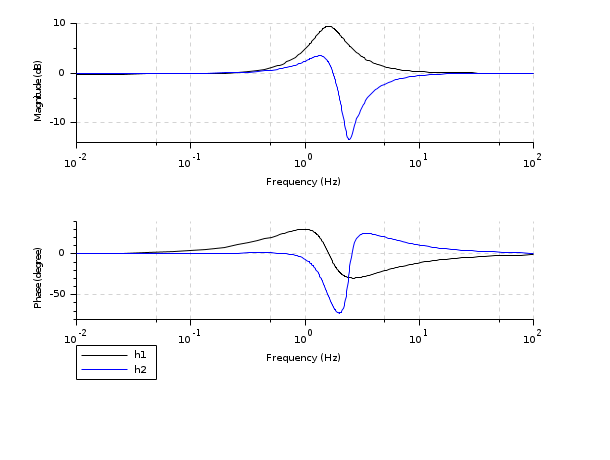Scilab Home page | Wiki | Bug tracker | Forge | Mailing list archives | ATOMS | File exchange
Change language to: Français - Português - 日本語 - Русский

# bode

Bode plot

### Syntax

```bode(sl, [fmin, fmax] [,step] [,comments] )

### Arguments

sl

A siso or simo linear dynamical system, in state space, transfer function or zpk representations, in continuous or discrete time.

fmin,fmax

real (frequency bounds (in Hz))

step

real (logarithmic step.)

vector of character strings (captions).

frq

row vector or matrix (frequencies (in Hz) ) (one row for each SISO subsystem).

db

row vector or matrix ( magnitudes (in Db)). (one row for each SISO subsystem).

phi

row vector or matrix ( phases (in degree)) (one row for each SISO subsystem).

repf

row vector or matrix of complex numbers (complex frequency response).

converts the frequency from Hz into rad/s (multiplies by 2π),

### Description

Bode plot, i.e magnitude and phase of the frequency response of the linear dynamical system `sl`.

`sl` can be a continuous-time or discrete-time SIMO system given by its state space, rational transfer function (see syslin) or zpk representation. In case of multi-output the outputs are plotted with different colors.

The frequencies are given by the bounds `fmin,fmax` (in Hz) or by a row-vector (or a matrix for multi-output) `frq`.

`step` is the ( logarithmic ) discretization step. (see `calfrq` for the choice of default value).

`comments` is a vector of character strings (captions).

`db,phi` are the matrices of modulus (in Db) and phases (in degrees). (One row for each response).

`repf` matrix of complex numbers. One row for each response.

Default values for `fmin` and `fmax` are `1.d-3`, `1.d+3` if `sl` is continuous-time or `1.d-3`, `0.5`/sl.dt (nyquist frequency) if `sl` is discrete-time. Automatic discretization of frequencies is made by `calfrq`.

The datatips tool may be used to display data along the phase and modulus curves.

### Examples

```s = poly(0, 's');
h = syslin('c', (s^2+2*0.9*10*s+100)/(s^2+2*0.3*10.1*s+102.01));

clf(); bode(h, 0.01, 100);``````s = poly(0, 's');
h1 = syslin('c', (s^2+2*0.9*10*s+100)/(s^2+2*0.3*10.1*s+102.01));
num = 22801+4406.18*s+382.37*s^2+21.02*s^3+s^4;
den = 22952.25+4117.77*s+490.63*s^2+33.06*s^3+s^4;
h2 = syslin('c', num/den);

clf(); bode([h1; h2], 0.01, 100, ['h1'; 'h2']);``````s = %s;
G = (10*(s+3))/(s*(s+2)*(s^2+s+2)); // A rational matrix
sys = syslin('c', G); // A continuous-time linear system in transfer matrix representation.
f_min = .0001; f_max = 16; // Frequencies in Hz• bode_asymp — Bode plot asymptote
• black — Black-Nichols diagram of a linear dynamical system
• nyquist — nyquist plot
• gainplot — magnitude plot
• repfreq — frequency response
• g_margin — gain margin and associated crossover frequency
• p_margin — phase margin and associated crossover frequency
• calfrq — frequency response discretization
• phasemag — phase and magnitude computation
• datatips — Tool for placing and editing tips along the plotted curves.

### History

 Version Description 6.0 handling zpk representation